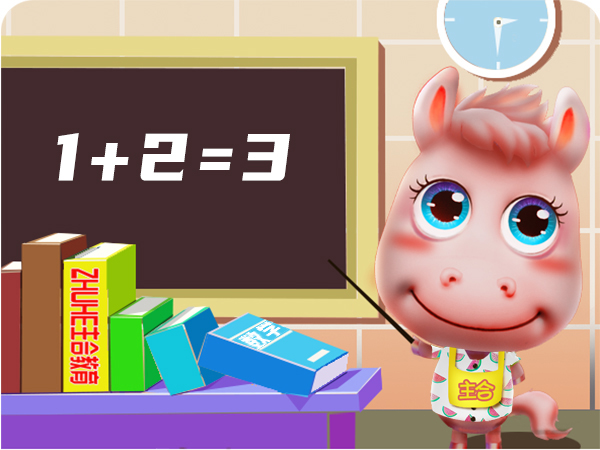# 【每日一题】小学数学1—6年级天天练习

1. 直接写出得数

54-4=     2+70=     50+30=

50-20=    77-7=      80+10=

2. 聪聪收集了58节废电池，丽丽收集了50节废电池，丽丽再收集多少节废电池才能与聪聪的一样多？3. 动物园里有棕熊、黑熊共15只，其中棕熊有5只，黑熊有多少只？

1. 算一算

56厘米+34厘米=（    ）分米

2米-8分米=（    ）分米

7100千克-100千克=（    ）吨

2. 笔算

548+237=    689+346=    310-207=

3. 在英语大赛的面试中，每次只能进去5名同学，乐乐的序号是26号，乐乐第几次进去？

1. 2000÷8的商的末尾有（     ）个0,702÷7的商的末尾有（     ）个0。

2. 估算

73÷8≈     142÷7≈     148÷5≈

3. 星星幼儿园准备买3辆童车，每辆童车的价格是297元，900元够吗？

1. 在横线上填上合适的单位名称。

(1)教室黑板的面积是420

(2)学校操场的面积大约是9600

(3)一个果园的面积大约是3

(4)天安门广场是世界上最大的广场,占地约40

2. 一块长方形玉米地,长是600米,宽是300米。如果每公顷平均收10吨玉米,那么这块玉米地能收多少吨玉米?

3. 小亮围着一个正方形校园走了1圈,一共3200米。这个校园的面积是多少公顷?

1. a和b都是自然数，分解质因数a=2×5×c，b=3×5×c，如果a和b的最大公因数是35，那么c=（    ），a和b的最小公倍数是（     ）。

2. 运动会上，操场一边每隔4米插一面彩旗（两端都有），一共插了31面，后来要减少一些彩旗，改成每隔6米插一面，有几面彩旗可以不用移动？

1. 在一幅比例尺是1：5000的图纸上，量得一块长方形菜地的长是4厘米，宽是1.2厘米。这块菜地的实际面积是多少平方米？

2. 一个圆锥形的谷堆高1.5米，底面直径是2米。如果每立方米的稻谷重650千克，这堆稻谷重多少千克？

1. 50   72   80；30   70   90

2. 58-50=8

3. 15-5=10（只）

1. 10   12   7

2. 785   1035   103

3. 26÷5=5（次）……1（名）

5+1=6（次）

1. 1   2

2. 9   20   30

3. 297≈300   300×3=900（元）够

1. 平方分米　平方米　公顷　公顷

2. 600×300=180000(平方米)

180000平方米=18公顷

18×10=180(吨)

3. 3200÷4=800(米)

800×800=640000(平方米)

640000平方米=64公顷

1. 7   210

2. （31-1）×4=120（米）

[4,6]=12   120÷12=10（段）

10+1=11（面）

1. 5000厘米=50米

50×4=200（米）50×1.2=60（米）

200×60=12000（平方米）

2. 2÷2=1（米）

1×1×3.14×1.5=4.71（立方米）

4.71×650=3061.5（千克）

▍标签：小学数学   每日一题

▍综合整理：小学数学

▍编辑：果果

--------------- 小学数学 ---------------